Home
Hostname: page-component-65d66dc8c9-q4bss Total loading time: 0.535 Render date: 2021-09-29T01:14:13.636Z Has data issue: true Feature Flags: { "shouldUseShareProductTool": true, "shouldUseHypothesis": true, "isUnsiloEnabled": true, "metricsAbstractViews": false, "figures": true, "newCiteModal": false, "newCitedByModal": true, "newEcommerce": true, "newUsageEvents": true }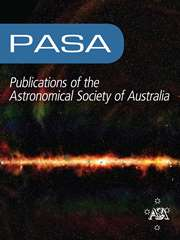Publications of the Astronomical Society of Australia

# The MAGPI survey: Science goals, design, observing strategy, early results and theoretical framework

Published online by Cambridge University Press:  26 July 2021

*
Author for correspondence: C. Foster, E-mail: caroline.foster@sydney.edu.au

## Abstract

We present an overview of the Middle Ages Galaxy Properties with Integral Field Spectroscopy (MAGPI) survey, a Large Program on the European Southern Observatory Very Large Telescope. MAGPI is designed to study the physical drivers of galaxy transformation at a lookback time of 3–4 Gyr, during which the dynamical, morphological, and chemical properties of galaxies are predicted to evolve significantly. The survey uses new medium-deep adaptive optics aided Multi-Unit Spectroscopic Explorer (MUSE) observations of fields selected from the Galaxy and Mass Assembly (GAMA) survey, providing a wealth of publicly available ancillary multi-wavelength data. With these data, MAGPI will map the kinematic and chemical properties of stars and ionised gas for a sample of 60 massive (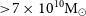${>}7 \times 10^{10} {\mathrm{M}}_\odot$ ) central galaxies at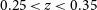$0.25 < z <0.35$ in a representative range of environments (isolated, groups and clusters). The spatial resolution delivered by MUSE with Ground Layer Adaptive Optics (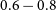$0.6-0.8$ arcsec FWHM) will facilitate a direct comparison with Integral Field Spectroscopy surveys of the nearby Universe, such as SAMI and MaNGA, and at higher redshifts using adaptive optics, for example, SINS. In addition to the primary (central) galaxy sample, MAGPI will deliver resolved and unresolved spectra for as many as 150 satellite galaxies at$0.25 < z <0.35$ , as well as hundreds of emission-line sources at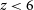$z < 6$ . This paper outlines the science goals, survey design, and observing strategy of MAGPI. We also present a first look at the MAGPI data, and the theoretical framework to which MAGPI data will be compared using the current generation of cosmological hydrodynamical simulations including EAGLE, Magneticum, HORIZON-AGN, and Illustris-TNG. Our results show that cosmological hydrodynamical simulations make discrepant predictions in the spatially resolved properties of galaxies at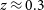$z\approx 0.3$ . MAGPI observations will place new constraints and allow for tangible improvements in galaxy formation theory.

Type
Research Article
© The Author(s), 2021. Published by Cambridge University Press on behalf of the Astronomical Society of Australia

## Access options

Get access to the full version of this content by using one of the access options below. (Log in options will check for institutional or personal access. Content may require purchase if you do not have access.)

## References

Arth, A., Dolag, K., Beck, A. M., Petkova, M., & Lesch, H. 2014, arXiv e-prints, p. arXiv:1412.6533Google Scholar
Bacon, R., et al. 2001, MNRAS, 326, 23Google Scholar
Bacon, R., et al. 2015, A&A, 575, A75Google Scholar
Bacon, R., et al. 2017, A&A, 608, A1Google Scholar
Baldry, I. K., et al. 2014, MNRAS, 441, 2440Google Scholar
Baldry, I. K., et al. 2018, MNRAS, 474, 3875Google Scholar
Baldwin, J. A., Phillips, M. M., & Terlevich, R. 1981, PASP, 93, 5CrossRefGoogle Scholar
Baugh, C. M., Cole, S., & Frenk, C. S. 1996, MNRAS, 282, L27CrossRefGoogle Scholar
Beifiori, A., Maraston, C., Thomas, D., & Johansson, J., 2011, A&A, 531, A109CrossRefGoogle Scholar
Bekki, K., & Couch, W. J. 2011, MNRAS, 415, 1783CrossRefGoogle Scholar
Belfiore, F., et al. 2017, MNRAS, 466, 2570Google Scholar
Bellstedt, S., et al. 2020, MNRAS, 496, 3235CrossRefGoogle Scholar
Bender, R., Saglia, R. P., & Gerhard, O. E. 1994, MNRAS, 269, 785CrossRefGoogle Scholar
Bershady, M. A., Verheijen, M. A. W., Swaters, R. A., Andersen, D. R., Westfall, K. B., & Martinsson, T. 2010, ApJ, 716, 198CrossRefGoogle Scholar
Bezanson, R., et al. 2018, ApJ, 858, 60CrossRefGoogle Scholar
Blanton, M. R., Eisenstein, D., Hogg, D. W., Schlegel, D. J., & Brinkmann, J. 2005, ApJ, 629, 143CrossRefGoogle Scholar
Bluck, A. F. L., et al. 2020, arXiv e-prints, p. arXiv:2009.05341Google Scholar
Borisova, E., et al. 2016, ApJ, 831, 39CrossRefGoogle Scholar
Boselli, A., & Gavazzi, G. 2006, PASP, 118, 517CrossRefGoogle Scholar
Brough, S., et al. 2013, MNRAS, 435, 2903Google Scholar
Bryant, J. J., et al. 2020, in Society of Photo-Optical Instrumentation Engineers (SPIE) Conference Series. p. 1144715, doi: 10.1117/12.2560309CrossRefGoogle Scholar
Bundy, K., et al. 2015, ApJ, 798, 7Google Scholar
Calzetti, D., Armus, L., Bohlin, R. C., Kinney, A. L., Koornneef, J., & Storchi-Bergmann, T. 2000, ApJ, 533, 682CrossRefGoogle Scholar
Cappellari, M. 2016, ARA&A, 54, 597CrossRefGoogle Scholar
Cappellari, M. 2017, MNRAS, 466, 798CrossRefGoogle Scholar
Cappellari, M., & Copin, Y. 2003, MNRAS, 342, 345CrossRefGoogle Scholar
Cappellari, M., & Emsellem, E. 2004, PASP, 116, 138CrossRefGoogle Scholar
Cappellari, M., et al. 2011, MNRAS, 413, 813Google Scholar
Carton, D., et al. 2018, MNRAS, 478, 4293CrossRefGoogle Scholar
Catelan, P., & Theuns, T. 1996, MNRAS, 282, 436CrossRefGoogle Scholar
Chabrier, G. 2003, PASP, 115, 763CrossRefGoogle Scholar
Choi, H., & Yi, S. K. 2017, ApJ, 837, 68CrossRefGoogle Scholar
Choi, H., Yi, S. K., Dubois, Y., Kimm, T., Devriendt, J. E. G., & Pichon, C. 2018, ApJ, 856, 114CrossRefGoogle Scholar
Collacchioni, F., Lagos, C. D. P., Mitchell, P. D., Schaye, J., Wisnioski, E., Cora, S. A., & Correa, C. A. 2020, MNRAS, 495, 2827CrossRefGoogle Scholar
Conroy, C., Villaume, A., van Dokkum, P. G., & Lind, K. 2018, ApJ, 854, 139CrossRefGoogle Scholar
Conselice, C. J. 2014, ARA&A, 52, 291CrossRefGoogle Scholar
Contini, T., et al. 2012, A&A, 539, A91Google Scholar
Cooper, M. C., et al. 2006, MNRAS, 370, 198Google Scholar
Cooper, M. C., et al. 2010, MNRAS, 409, 337Google Scholar
Cox, T. J., Dutta, S. N., Di Matteo, T., Hernquist, L., Hopkins, P. F., Robertson, B., & Springel, V. 2006, ApJ, 650, 791CrossRefGoogle Scholar
Crain, R. A., et al. 2015, MNRAS, 450, 1937Google Scholar
Croom, S. M., et al. 2012, MNRAS, 421, 872Google Scholar
Davies, L. J. M., et al. 2015, MNRAS, 452, 616Google Scholar
Davies, L. J. M., et al. 2018, MNRAS, 480, 768Google Scholar
Davies, L. J. M., et al. 2019, MNRAS, 483, 5444Google Scholar
Davies, J. J., Crain, R. A., & Pontzen, A. 2020, arXiv e-prints, p. arXiv:2006.13221Google Scholar
Davies, R. L., Kewley, L. J., Ho, I. T., & Dopita, M. A. 2014, MNRAS, 444, 3961CrossRefGoogle Scholar
Deeley, S., et al. 2017, MNRAS, 467, 3934CrossRefGoogle Scholar
De Lucia, G., Springel, V., White, S. D. M., Croton, D., & Kauffmann, G. 2006, MNRAS, 366, 499CrossRefGoogle Scholar
de Zeeuw, P. T., et al. 2002, MNRAS, 329, 513CrossRefGoogle Scholar
Dolag, K., Jubelgas, M., Springel, V., Borgani, S., & Rasia, E. 2004, ApJ, 606, L97CrossRefGoogle Scholar
Dolag, K., Mevius, E., & Remus, R.-S. 2017, Galaxies, 5, 35Google Scholar
Dopita, M. A., & Sutherland, R. S. 2003, Astrophysics of the diffuse universeCrossRefGoogle Scholar
Dressler, A. 1980, ApJ, 236, 351CrossRefGoogle Scholar
Driver, S. P., Windhorst, R. A., & Griffiths, R. E. 1995b, ApJ, 453, 48CrossRefGoogle Scholar
Driver, S. P., Windhorst, R. A., Ostrander, E. J., Keel, W. C., Griffiths, R. E., & Ratnatunga, K. U. 1995a, ApJ, 449, L23CrossRefGoogle Scholar
Driver, S. P., et al. 2011, MNRAS, 413, 971Google Scholar
Driver, S. P., et al. 2016, MNRAS, 455, 3911Google Scholar
Dubois, Y., Peirani, S., Pichon, C., Devriendt, J., Gavazzi, R., Welker, C., & Volonteri, M. 2016, MNRAS, 463, 3948CrossRefGoogle Scholar
Emsellem, E., et al. 2007, MNRAS, 379, 401Google Scholar
Emsellem, E., et al. 2011, MNRAS, 414, 888Google Scholar
Erroz-Ferrer, S., et al. 2019, MNRAS, 484, 5009CrossRefGoogle Scholar
Fabian, A. C. 2012, ARA&A, 50, 455CrossRefGoogle Scholar
Förster Schreiber, N. M., et al. 2018, ApJS, 238, 21Google Scholar
Gaia Collaboration, et al. 2018, A&A, 616, A1Google Scholar
Glazebrook, K., Ellis, R., Santiago, B., & Griffiths, R., 1995, MNRAS, 275, L19CrossRefGoogle Scholar
Graham, M. T., et al. 2018, MNRAS, 477, 4711Google Scholar
Guérou, A., et al. 2017, A&A, 608, A5CrossRefGoogle Scholar
Harborne, K. E., Power, C., & Robotham, A. S. G. 2020, PASA, 37, e016CrossRefGoogle Scholar
Hemler, Z. S., et al. 2020, arXiv e-prints, p. arXiv:2007.10993Google Scholar
Herenz, E. C., et al. 2017, A&A, 606, A12CrossRefGoogle Scholar
Herenz, E. C., et al. 2019, A&A, 621, A107CrossRefGoogle Scholar
Hinshaw, G., et al. 2013, ApJS, 208, 19CrossRefGoogle Scholar
Ho, I. T., Kudritzki, R.-P., Kewley, L. J., Zahid, H. J., Dopita, M. A., Bresolin, F., & Rupke, D. S. N. 2015, MNRAS, 448, 2030CrossRefGoogle Scholar
Kassin, S. A., et al. 2012, ApJ, 758, 106CrossRefGoogle Scholar
Kauffmann, G. 1995, MNRAS, 274, 153CrossRefGoogle Scholar
Kauffmann, G., White, S. D. M., Heckman, T. M., Ménard, B., Brinchmann, J., Charlot, S., Tremonti, C., & Brinkmann, J. 2004, MNRAS, 353, 713CrossRefGoogle Scholar
Kelvin, L. S., et al. 2012, MNRAS, 421, 1007Google Scholar
Kewley, L. J., Groves, B., Kauffmann, G., & Heckman, T. 2006, MNRAS, 372, 961CrossRefGoogle Scholar
Kewley, L. J., Nicholls, D. C., & Sutherland, R. S. 2019, ARA&A, 57, 511CrossRefGoogle Scholar
Khochfar, S., et al. 2011, MNRAS, 417, 845Google Scholar
Kobayashi, C. 2004, MNRAS, 347, 740CrossRefGoogle Scholar
Kobayashi, C., Karakas, A. I., & Umeda, H. 2011, MNRAS, 414, 3231CrossRefGoogle Scholar
Komatsu, E., et al. 2011, ApJS, 192, 18CrossRefGoogle Scholar
Krajnović, D., et al. 2011, MNRAS, 414, 2923Google Scholar
Krajnović, D., et al. 2020, A&A, 635, A129Google Scholar
Kreckel, K., et al. 2019, ApJ, 887, 80CrossRefGoogle Scholar
Krumholz, M. R., & Ting, Y.-S. 2018, MNRAS, 475, 2236CrossRefGoogle Scholar
Lagattuta, D. J., et al. 2019, MNRAS, 485, 3738Google Scholar
Lagos, C. d. P., et al. 2018a, MNRAS, 473, 4956Google Scholar
Lagos, C. d. P., Schaye, J., Bahé, Y., Van de Sande, J., Kay, S. T., Barnes, D., Davis, T. A., & Dalla Vecchia, C. 2018b, MNRAS, 476, 4327CrossRefGoogle Scholar
Lagos, C. d. P., Theuns, T., Stevens, A. R. H., Cortese, L., Padilla, N. D., Davis, T. A., Contreras, S., & Croton, D. 2017, MNRAS, 464, 3850CrossRefGoogle Scholar
Liske, J., et al. 2015, MNRAS, 452, 2087Google Scholar
Liu, Y., et al. 2016, ApJ, 818, 179Google Scholar
López-Sanjuan, C., et al. 2015, A&A, 576, A53Google Scholar
Ludlow, A. D., Schaye, J., Schaller, M., & Bower, R. 2020, MNRAS, 493, 2926CrossRefGoogle Scholar
Ma, C.-P., Greene, J. E., McConnell, N., Janish, R., Blakeslee, J. P., Thomas, J., & Murphy, J. D. 2014, ApJ, 795, 158CrossRefGoogle Scholar
Ma, X., Hopkins, P. F., Feldmann, R., Torrey, P., Faucher-Giguère, C.-A., & Kereš, D. 2017, MNRAS, 466, 4780Google Scholar
Magdis, G. E., et al. 2016, MNRAS, 456, 4533CrossRefGoogle Scholar
Mahler, G., et al. 2018, MNRAS, 473, 663CrossRefGoogle Scholar
Maiolino, R., et al. 2008, A&A, 488, 463Google Scholar
Man, A., & Belli, S. 2018, NatAs, 2, 695CrossRefGoogle Scholar
McAlpine, S., et al. 2016, A&C, 15, 72Google Scholar
Mitchell, P. D., Schaye, J., Bower, R. G., & Crain, R. A. 2020, MNRAS, 494, 3971CrossRefGoogle Scholar
Moran, S. M., Ellis, R. S., Treu, T., Smith, G. P., Rich, R. M., & Smail, I. 2007, ApJ, 671, 1503CrossRefGoogle Scholar
Mundy, C. J., Conselice, C. J., Duncan, K. J., Almaini, O., Häußler, B., & Hartley, W. G. 2017, MNRAS, 470, 3507CrossRefGoogle Scholar
Naab, T., & Ostriker, J. P. 2017, ARA&A, 55, 59CrossRefGoogle Scholar
Naab, T., et al. 2014, MNRAS, 444, 3357Google Scholar
Naiman, J. P., et al. 2018, MNRAS, 477, 1206CrossRefGoogle Scholar
Nelson, D., et al. 2019, MNRAS, 490, 3234CrossRefGoogle Scholar
Newman, A. B., Belli, S., Ellis, R. S., & Patel, S. G. 2018, ApJ, 862, 126CrossRefGoogle Scholar
Oh, S., et al. 2018, ApJS, 237, 14CrossRefGoogle Scholar
Oh, S., et al. 2019, MNRAS, 488, 4169CrossRefGoogle Scholar
Oke, J. B., & Gunn, J. E. 1983, ApJ, 266, 713CrossRefGoogle Scholar
Papovich, C., et al. 2018, ApJ, 854, 30CrossRefGoogle Scholar
Peng, Y.-j. 2010, ApJ, 721, 193Google Scholar
Penoyre, Z., Moster, B. P., Sijacki, D., & Genel, S. 2017, MNRAS, 468, 3883CrossRefGoogle Scholar
Pilkiene, M., Alonderiene, R., Chmieliauskas, A., Simkonis, S., & Muller, R. 2018, IJPM, 36, 913CrossRefGoogle Scholar
Pillepich, A., et al. 2018, MNRAS, 473, 4077CrossRefGoogle Scholar
Planck Collaboration 2014, A&A, 571, A16Google Scholar
Planck Collaboration, et al. 2016, A&A, 594, A13Google Scholar
Poetrodjojo, H., et al. 2021, MNRAS, 502, 3357CrossRefGoogle Scholar
Poggianti, B. M., et al. 2017, ApJ, 844, 48CrossRefGoogle Scholar
Queyrel, J., et al. 2012, A&A, 539, A93CrossRefGoogle Scholar
Robotham, A. S. G. 2018, ProFound: Source Extraction and Application to Modern Survey Data (ascl:1804.006)Google Scholar
Robotham, A. S. G., et al. 2011, MNRAS, 416, 2640Google Scholar
Robotham, A. S. G., et al. 2014, MNRAS, 444, 3986Google Scholar
Robotham, A. S. G., Davies, L. J. M., Driver, S. P., Koushan, S., Taranu, D. S., Casura, S., & Liske, J. 2018, MNRAS, 476, 3137CrossRefGoogle Scholar
Rodriguez-Gomez, V., et al. 2015, MNRAS, 449, 49CrossRefGoogle Scholar
Salpeter, E. E. 1955, ApJ, 121, 161CrossRefGoogle Scholar
Sánchez-Menguiano, L., et al. 2016, A&A, 587, A70Google Scholar
Sánchez, S. F., et al. 2012, A&A, 538, A8Google Scholar
Sarzi, M., et al. 2018, A&A, 616, A121CrossRefGoogle Scholar
Schaefer, A. L., et al. 2019, MNRAS, 483, 2851Google Scholar
Schaye, J., & Dalla Vecchia, C. 2008, MNRAS, 383, 1210CrossRefGoogle Scholar
Schaye, J., et al. 2015, MNRAS, 446, 521Google Scholar
Schulze, F., Remus, R.-S., Dolag, K., Burkert, A., Emsellem, E., & van de Ven, G. 2018, MNRAS, 480, 4636CrossRefGoogle Scholar
Scott, N., et al. 2017, MNRAS, 472, 2833Google Scholar
Sharda, P., Krumholz, M. R., Wisnioski, E., Acharyya, A., Federrath, C., & Forbes, J. C. 2021, MNRAS, 504, 53CrossRefGoogle Scholar
Simons, R. C., et al. 2017, ApJ, 843, 46CrossRefGoogle Scholar
Skibba, R. A., et al. 2009, MNRAS, 399, 966CrossRefGoogle Scholar
Soto, K. T., Lilly, S. J., Bacon, R., Richard, J., & Conseil, S. 2016, MNRAS, 458, 3210CrossRefGoogle Scholar
Sparre, M., & Springel, V. 2017, MNRAS, 470, 3946CrossRefGoogle Scholar
Spitzer Lyman, J. 1962, AS, 50, 473Google Scholar
Springel, V., & Hernquist, L. 2003, MNRAS, 339, 289CrossRefGoogle Scholar
Springel, V., et al. 2018, MNRAS, 475, 676CrossRefGoogle Scholar
Stott, J. P., et al. 2014, MNRAS, 443, 2695CrossRefGoogle Scholar
Stott, J. P., et al. 2016, MNRAS, 457, 1888CrossRefGoogle Scholar
Sturch, L. K., & Madore, B. F. 2012, in IAU Symposium Vol. 284, The Spectral Energy Distribution of Galaxies- SED 2011, ed. Tuffs, R. J., & Popescu, C. C., 180, doi: 10.1017/S1743921312009027CrossRefGoogle Scholar
Sutherland, R. S., & Dopita, M. A. 1993, ApJS, 88, 253CrossRefGoogle Scholar
Taranu, D. S., Dubinski, J., & Yee, H. K. C. 2013, ApJ, 778, 61CrossRefGoogle Scholar
Taylor, P., & Kobayashi, C. 2015, MNRAS, 448, 1835CrossRefGoogle Scholar
Taylor, P., & Kobayashi, C. 2017, MNRAS, 471, 3856CrossRefGoogle Scholar
Taylor, E. N., et al. 2011, MNRAS, 418, 1587Google Scholar
Teklu, A. F., Remus, R.-S., Dolag, K., Beck, A. M., Burkert, A., Schmidt, A. S., Schulze, F., & Steinborn, L. K. 2015, ApJ, 812, 29CrossRefGoogle Scholar
Thomas, D., Maraston, C., Bender, R., & Mendes de Oliveira, C. 2005, ApJ, 621, 673CrossRefGoogle Scholar
Thomas, D., Maraston, C., Schawinski, K., Sarzi, M., & Silk, J. 2010, MNRAS, 404, 1775Google Scholar
Tiley, A. L., et al. 2020, MNRAS, 496, 649CrossRefGoogle Scholar
Tissera, P. B., Machado, R. E. G., Carollo, D., Minniti, D., Beers, T. C., Zoccali, M., & Meza, A. 2018, MNRAS, 473, 1656CrossRefGoogle Scholar
Tissera, P. B., Rosas-Guevara, Y., Bower, R. G., Crain, R. A., del P Lagos, C., Schaller, M., Schaye, J., & Theuns, T. 2019, MNRAS, 482, 2208CrossRefGoogle Scholar
Toft, S., et al. 2017, doi: 10.1038/nature22388, 546, 510CrossRefGoogle Scholar
Trayford, J. W., & Schaye, J. 2019, MNRAS, 485, 5715CrossRefGoogle Scholar
Trujillo, I., & Pohlen, M. 2005, ApJ, 630, L17CrossRefGoogle Scholar
Turner, O. J., et al. 2017, MNRAS, 471, 1280Google Scholar
Übler, H., et al. 2019, ApJ, 880, 48Google Scholar
Urrutia, T., et al. 2019, A&A, 624, A141Google Scholar
Valdes, F., Gupta, R., Rose, J. A., Singh, H. P., & Bell, D. J. 2004, ApJS, 152, 251CrossRefGoogle Scholar
van der Marel, R. P., & van Dokkum, P. G. 2007, ApJ, 668, 738CrossRefGoogle Scholar
van der Velden, E. 2020, JOSS, 5, 2004CrossRefGoogle Scholar
van der Wel, A., Bell, E. F., Holden, B. P., Skibba, R. A., & Rix, H.-W. 2010, ApJ, 714, 1779CrossRefGoogle Scholar
van der Wel, A., & van der Marel, R. P. 2008, ApJ, 684, 260CrossRefGoogle Scholar
van der Wel, A., et al. 2014, ApJ, 788, 28Google Scholar
van der Wel, A., et al. 2016, ApJS, 223, 29CrossRefGoogle Scholar
van de Sande, J., et al. 2017, ApJ, 835Google Scholar
van de Sande, J., et al. 2019, MNRAS, 484, 869Google Scholar
van de Sande, J., et al. 2020, arXiv e-prints, p. arXiv:2011.08199Google Scholar
Vaughan, S. P., et al. 2020, MNRAS, 496, 3841CrossRefGoogle Scholar
Vazdekis, A., et al. 2015, MNRAS, 449, 1177CrossRefGoogle Scholar
Veilleux, S., & Osterbrock, D. E. 1987, ApJS, 63, 295CrossRefGoogle Scholar
Vogelsberger, M., Marinacci, F., Torrey, P., & Puchwein, E. 2020, NRP, 2, 42CrossRefGoogle Scholar
Walo-Martín, D., Falcón-Barroso, J., Dalla Vecchia, C., Pérez, I., & Negri, A. 2020, MNRAS, 494, 5652CrossRefGoogle Scholar
Wang, B., Cappellari, M., Peng, Y., & Graham, M. 2020, MNRAS, 495, 1958CrossRefGoogle Scholar
Weilbacher, P. M., Streicher, O., Urrutia, T., Jarno, A., Pécontal-Rousset, A., Bacon, R., & Böhm, P. 2012, in Proceedings of SPIE. p. 84510B, doi: 10.1117/12.925114CrossRefGoogle Scholar
Weilbacher, P. M., et al. 2020, arXiv e-prints, p. arXiv:2006.08638Google Scholar
Wiersma, R. P. C., Schaye, J., Theuns, T., Dalla Vecchia, C., & Tornatore, L. 2009, MNRAS, 399, 574CrossRefGoogle Scholar
Wisnioski, E., et al. 2015, ApJ, 799, 209Google Scholar
Wisnioski, E., et al. 2019, ApJ, 886, 124Google Scholar
Wright, R. J., Lagos, C. d. P., Power, C., &Mitchell, P. D. 2020, MNRAS,Google Scholar
Wuyts, S., et al. 2011, ApJ, 742, 96Google Scholar
Wuyts, E., et al. 2016, ApJ, 827, 74CrossRefGoogle Scholar
Yang, Y., et al. 2008, A&A, 477, 789Google Scholar
Yuan, T. T., Kewley, L. J., Swinbank, A. M., & Richard, J. 2012, ApJ, 759, 66CrossRefGoogle Scholar
Zurita, A., Florido, E., Bresolin, F., Pérez, I., & Pérez-Montero, E. 2021, MNRAS, 500, 2380CrossRefGoogle Scholar

# Send article to Kindle

Note you can select to send to either the @free.kindle.com or @kindle.com variations. ‘@free.kindle.com’ emails are free but can only be sent to your device when it is connected to wi-fi. ‘@kindle.com’ emails can be delivered even when you are not connected to wi-fi, but note that service fees apply.

Find out more about the Kindle Personal Document Service.

The MAGPI survey: Science goals, design, observing strategy, early results and theoretical framework
Available formats
×

# Send article to Dropbox

To send this article to your Dropbox account, please select one or more formats and confirm that you agree to abide by our usage policies. If this is the first time you use this feature, you will be asked to authorise Cambridge Core to connect with your <service> account. Find out more about sending content to Dropbox.

The MAGPI survey: Science goals, design, observing strategy, early results and theoretical framework
Available formats
×

# Send article to Google Drive

To send this article to your Google Drive account, please select one or more formats and confirm that you agree to abide by our usage policies. If this is the first time you use this feature, you will be asked to authorise Cambridge Core to connect with your <service> account. Find out more about sending content to Google Drive.

The MAGPI survey: Science goals, design, observing strategy, early results and theoretical framework
Available formats
×
×